(in WR FranklinResearch)

# Intro

Nearpt3 is a pair of subroutines to find nearest points in E3. Preprocess takes a list of fixed points, and preprocesses them into a data structure. Query takes a query point, and returns the closest fixed point to it.

Nearpt3 is very fast, very small, and can process very nonhomogeneous data. The largest example run on a laptop computer was St Matthew from the Stanford Digital Michelangelo Project Archive, with 184,088,599 fixed points.

Preprocessing the David data set, with 28,158,109 fixed points, into a 7233 grid took 0.6 microseconds per fixed point. Each query required 6 microseconds. The platform was an IBM T43p laptop computer with a 2GHz Intel Pentium M processor and 2GB of memory.

Please see this reasonably complete draft paper describing Nearpt3. It gives test results on the following 16 datasets, shown as unstructured point clouds, which is how I used them.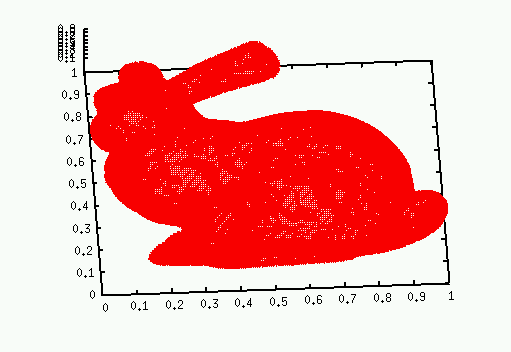Bunny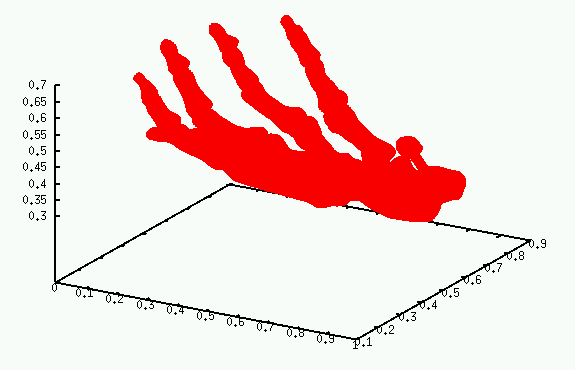Hand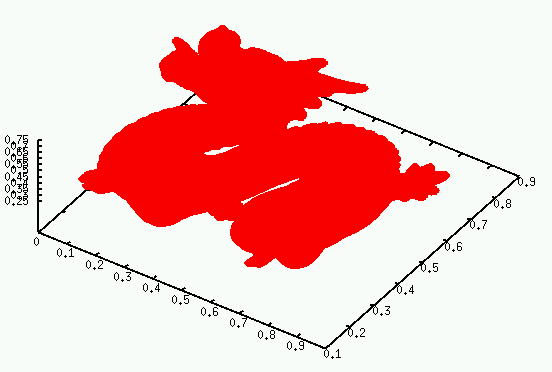Dragon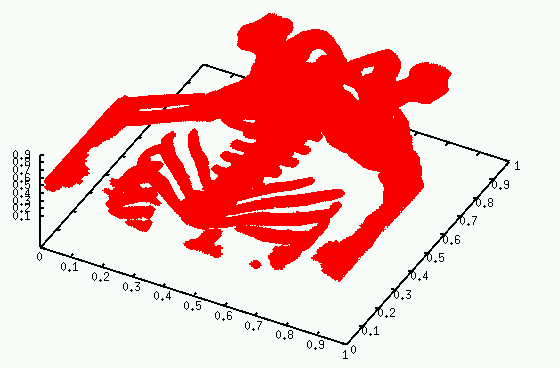Bone6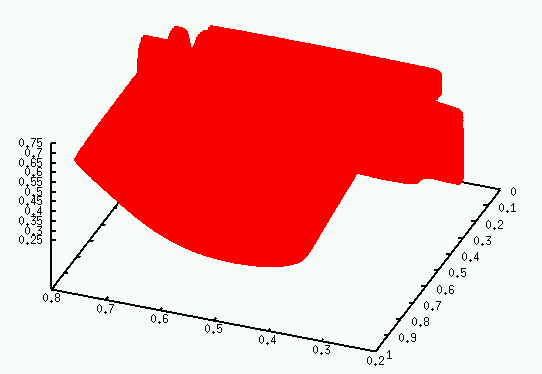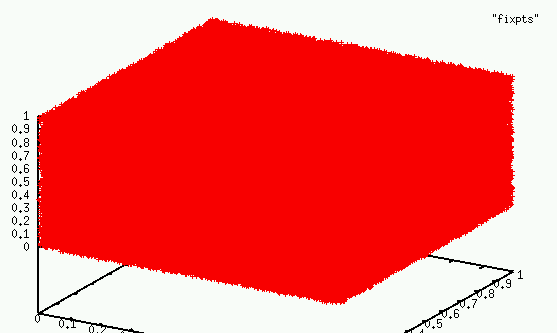Uniform i.i.d. with 100K, 300K,
1M, 3M, 10M, 30M, or 100M Points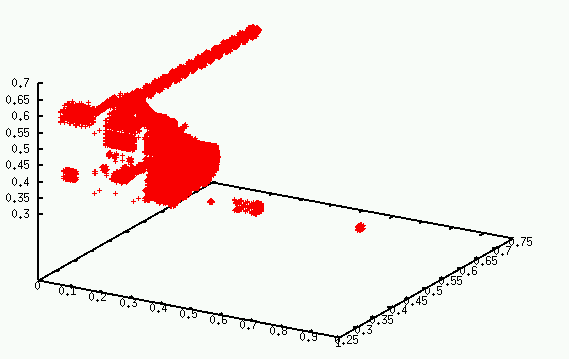UNC Complete Powerplant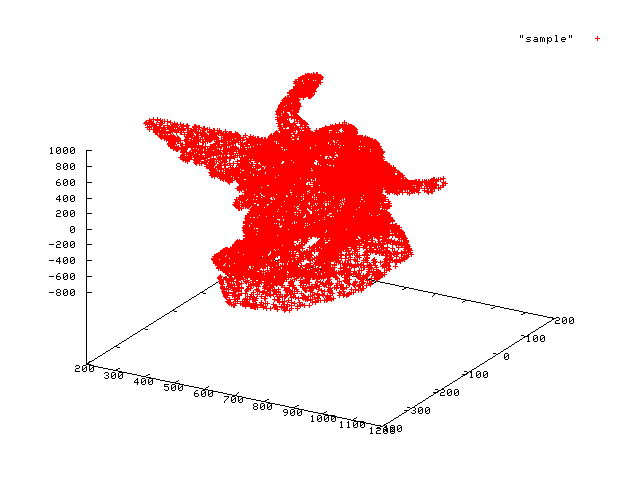Lucy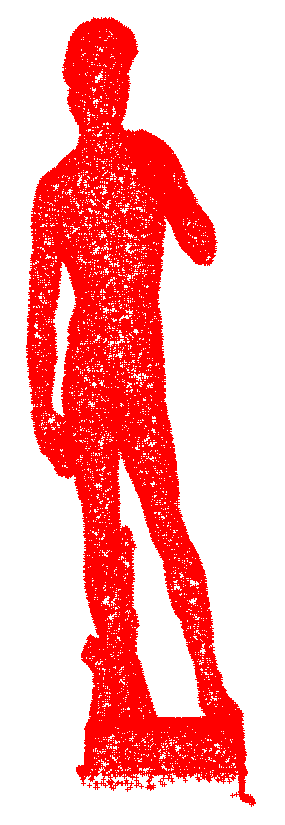David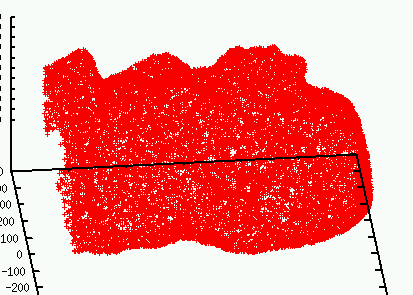St Matthew

The data had the following sources.

The paper also covers these topics:

1. Tradeoff between grid size and speed.
2. Distribution of fixed points per grid cell for two datasets:
1. a uniform distribution of 108 points.
2. Michelangelo's David, with 28,158,109 fixed points. The points of this dataset are quite evenly distributed, with a local topology that is often two dimensional.
3. Distribution of the number of cells searched per query point for the same two datasets.
4. Comparison to ANN (Approximate Nearest Neighbors).

Details not appropriate for the paper follow on this web page.

# Nearpt3 Usage

1. Nearpt3 is templated on the coordinate type, so decide what type your coordinates will be, say float. (However, each point's coordinates are converted to double before any operations, like computing which cell contains it. Therefore types like rational or bignum would require editing the source.)
2. Nearpt3 uses the Boost array class.
3. Read your nfixpts fixed points into a standard C array, p whose elements are boost::array<float,3>.
4. Declare a grid variable to hold the preprocessed data, and preprocess the fixed points into it:

nearpt3::Grid_T<float> *g = nearpt3::Preprocess(nfixpts, p);

5. Read a query point, q, of type boost:array<float,3>.
6. Make a query:

int closestpt = nearpt3::Query(g, q);

Note that closestpt is the index in p of the closest point.

7. Repeat as desired.

# Program Versions

The tarball has some different main programs to call Preprocess and Query, which are in the file nearpt3.cc.

1. main5.cc

This is the smallest sample main program to demonstrate nearpt3.

1. main5 reads fixed points as 3 floats from cin ...
2. terminated by a single number <= -1e30.
3. Then main5 calls Preprocess with a default value of ng_factor.
4. Next it reads query points from cin and for each, finds the closest fixed point.
2. main7.cc

This is the standard version used in my time tests.

1. main7 requires one float command line arg: ng_factor. If in doubt, use 1.
2. main7 reads fixed points from file fixpts.
3. main7 reads queries from file qpts. Both files contain triples of binary unsigned short ints.
4. Why binary? Reading ascii files would take more time than the whole rest of nearpt3's computations.
5. main7 writes results to binary file pairs. Each result is a query followed by the nearest fixed point, both as triples of unsigned short int coords.
6. main7 writes a line of statistics preceded by a line of documentation. The line of statistics is formatted for inclusion in a LaTeX table.
7. If macro EXHAUSTIVECHECK is defined when main7 is compiled, then each query result is exhaustively checked against all the fixed points to see whether it is really the closest. This can be very slow.
8. If macro STATS is defined when main7 is compiled, then extra statistics are computed and printed. They include histograms of the number of fixed points per grid cell and cells searched per query.

# Notes

1. The input points must be normalized to the range [0,1].
2. If the fixed points are uniformly distributed, ng_factor=0.5 was optimal in my tests. If they are unevenly distributed, then ng_factor=1 or higher, is better.
3. If two fixed points are equally close to the query, then Nearpt3 returns the one with smallest index.
4. You may compute and query several sets of fixed points, stored in different grid variables, simultaneously.
5. However, you must not change p, the array of fixed points while querying, since the grid doesn't duplicatively store the points, but stores &p.
6. The following files and utility programs are included in the tarball:
1. nearpt3.cc The nearpt3 classes and subroutines.
2. The various main programs listed above.
3. compcellsearchorder.cc computes the file cellsearchorder that nearpt3.cc includes when being compiled. Generally, I use only the first 30,000 lines of cellsearchorder.
4. cellsearchorder
5. makerandpts.cc generates i.i.d. random points in [0,1] forever. I used, e.g.,

makerandpts | head -1000000

to make the uni1m test data.

6. splitfq2.cc reads ASCII file points and writes BINARY files fixpts and qpts. The binary files have 6 bytes per point, for 3 unsigned short ints.
7. asc2binpts.cc Read ASCII points and write in BINARY.
8. printdist.cc Read a pairs file and print the distances between the queries and answers.
9. One way to plot the data, if points is ascii:

gnuplotsplot "points"

and capture the output with xv
10. This version of nearpt3 is much faster than earlier versions, particularly on nonuniform data.

# Availability

Nearpt3 is freely available for nonprofit research and education. I would appreciate an acknowledgement, and a link to my homepage.

# Nearpt2

Nearpt2, a special case of Nearpt3 is an online program to find nearest points in 2D. It reads a list of points, and preprocesses them into a data structure. Then it reads a list of query points, and, one by one, finds the closest fixed point to each query point.

Nearpt2 is very fast and very small. One test used vertices of two nonuniform meshes from Mark Shepherd. Processing the 10267 fixed points took about 0.01 CPU seconds on a 1600 MHz Pentium. Querying all 8005 test points took about 0.02 seconds.

Another test used 10,000,000 uniformly randomly generated fixed points and another 10,000,000 query points. The total preprocessing time was 12 CPU seconds on my laptop. The total query time was 65 seconds. The virtual memory was 460MB. The elapsed time including the main program's I/O was 415 seconds. (2003).